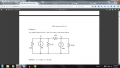# FIND V1 AND V2 USING NODAL ANALYSIS

#### HPage5

Joined Jan 17, 2016
15
Hi all.

Its late and I am stuck on a problem. I will attach a screen shot for ease.

I slightly understand mesh, but nodal confuses the crap out of me.

Whats throwing me is that there are 2 current sources inside the resistors.

I keep trying to find my equations to solve for V1 and V2, but I end up with 8V and 6V, when it should be 2 and 8.
And I am not sure where I am going wrong.

The two resistors in the middle, in series with each other---can I, or should I even, combine the two to make one equivalent resistor?
Will that make the solving easier, or more complicated?

Any help/advice is greatly appreciated. thanks.#### RBR1317

Joined Nov 13, 2010
706
Working with mesh currents is confusing, but writing node equations is so simple you don't even need to think about it. With no thinking there is nothing to be confused about. The node equation implicitly applies Kirchhoff's current law - the sum of currents at the node must be zero. Currents are expressed in terms of the node voltages. Each node equation is a sum of terms, each term is formed as follows: (Node Voltage - adjacent Node Voltage)/(Resistance between those two nodes). For current sources, current leaving the node is positive. Do that for all adjacent nodes and current sources, and that is the node equation.

For example, node #1 in Problem 1 has the following node equation: (let the bottom rail be ground - zero volts)

(V1-0)/2 +(4) +(V1-0)/2 +(V1-V2)/1 =0

See if you can write the node equation for node #2, then solve for V1 & V2.